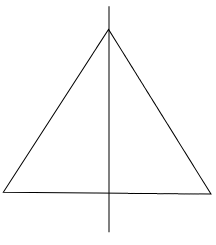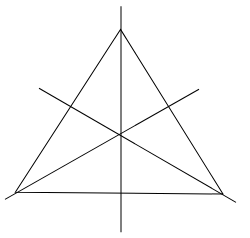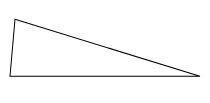# Can you draw a triangle which has(a) exactly one line of symmetry?(b) exactly two lines of symmetry?(c) exactly three lines of symmetry?(d) no lines of symmetry?Sketch a rough figure in each case.

To do:

We have to draw a triangle which has

(a) exactly one line of symmetry

(b) exactly two lines of symmetry

(c) exactly three lines of symmetry

(d) no lines of symmetry

Solution:

(a) We can draw an isosceles triangle which has only 1 line of symmetry as shown in the below figure.(b) We cannot draw a triangle which has 2 lines of symmetry.

(c) We can draw an equilateral triangle which has 3 lines of symmetry as shown in the below figure.(d) We can draw a scalene triangle which has no line of symmetry as shown in the below figure.Updated on: 10-Oct-2022

34 Views